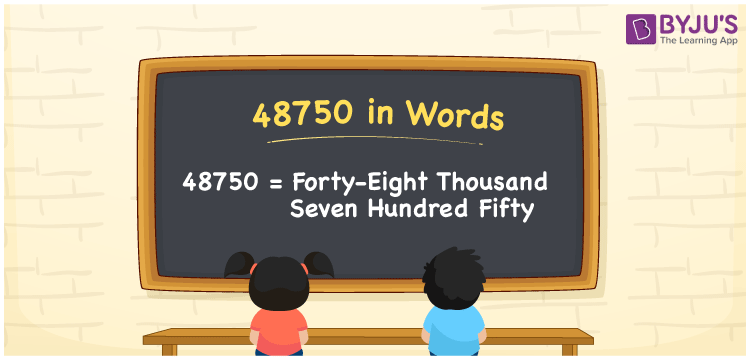# 48750 in Words

48750 in words is written as Forty-Eight Thousand Seven Hundred Fifty. For instance, Ajay purchased a new laptop worth Rs. 48750, then you can express the same sentence in words as “Ajay purchased a new laptop worth Rupees Forty-Eight Thousand Seven Hundred Fifty”. 48750 is a cardinal number or counting number. This article explains how to derive a numerical name with the help of a place value chart.

 48750 in Words Forty-Eight Thousand Seven Hundred Fifty Forty-Eight Thousand Seven Hundred Fifty in numerical form 48750

## 48750 in English Words

Generally, we write numbers in words using the English alphabet as it is a widely used language. Therefore, we can write and read the number 48750 in English as Forty-Eight Thousand Seven Hundred Fifty.## How to Write 48750 in Words?

We can write the number name for the given number easily by identifying the place value of the number. 48750 is a five-digit number. Hence, let us make a chart of 5 columns.

 Ten Thousand Thousand Hundreds Tens Ones 4 8 7 5 0

Therefore, we can write the expanded form as:

4 x Ten Thousand + 8 x Thousand + 7 x Hundred + 5 x Ten + 0 x One

= 4 x 10000 + 8 x 1000 + 7 x 100 + 5 x 10 + 0 x 1

= 40000 + 8000 + 700 + 50 + 0

= 40000 + 8000 + 700 + 50

= 48750

= Forty-Eight Thousand Seven Hundred Fifty

Hence, 48750 in words is written as Forty-Eight Thousand Seven Hundred Fifty

Interesting way of writing 48750 in words

4 = Four

48 = Forty-Eight

487 = Four Hundred and Eighty-Seven

4875 = Four Thousand Eight Hundred Seventy-Five

48750 = Forty-Eight Thousand Seven Hundred Fifty

Thus, the word form of the number 48750 is Forty-Eight Thousand Seven Hundred Fifty

48750 is a natural number that is the successor of 48749 and the predecessor of 48751

• 48750 in words – Forty-Eight Thousand Seven Hundred Fifty
• Is 48750 an odd number? – No
• Is 48750 an even number? – Yes
• Is 48750 a perfect square number? – No
• Is 48750 a perfect cube number? – No
• Is 48750 a prime number? – No
• Is 48750 a composite number? – Yes

## Frequently Asked Questions on 48750 in Words

Q1

### How to write 48750 in words?

48750 in words is written as Forty-Eight Thousand Seven Hundred Fifty.
Q2

### Simplify 40000 + 8750, and express in words.

Simplifying 40000 + 8750, we get 48750. 48750 in words is written as Forty-Eight Thousand Seven Hundred Fifty.
Q3

### 48750 is an even number. True or False.

Yes, the number 48750 is an even number.